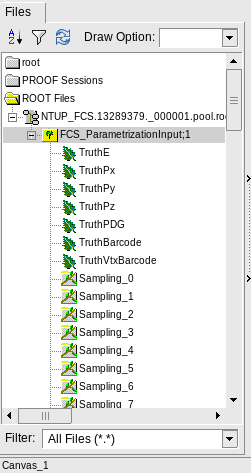ROOT Version: Not Provided
Platform: Not Provided
Compiler: Not Provided

I am trying to read out values from a ROOT file using PyROOT and is stuck with this issue. Any help is appreciated.

I based my code on this link. I think I am following everything as is given in that example. However, instead of it reading out momentum values as it is supposed to, I am getting a constant output of zeros. My tree structure is given as in the figure as shown by TBrowser.

``````import ROOT
rootFile = "file.root"

tree = f.Get('FCS_ParametrizationInput')
leaves = tree.GetListOfLeaves()

# define dynamically a python class containing root Leaves objects
class PyListOfLeaves(dict) :
pass

# create an istance
pyl = PyListOfLeaves()

for i in range(0,leaves.GetEntries() ) :
leaf = leaves.At(i)
name = leaf.GetName()
# add dynamically attribute to my class
pyl.__setattr__(name,leaf)

if name == 'TruthPz':
break

nev = tree.GetEntries()
for iev in range(0,nev) :
tree.GetEntry(iev)
# get values from the tree using Python class pyl which contains leaves
# objects
px = pyl.TruthPx.GetValue()
py = pyl.TruthPy.GetValue()
pz = pyl.TruthPz.GetValue()

print(px)
if iev == 10:
break
```````PyAIDA` is super ancient and completely deprecated (since… 2005, give or take).

something like this ought to work:

``````import ROOT
fname = "file.root"

f = ROOT.TFile.Open(fname)
tree = f.Get("FCS_ParametrizationInput")

n = 0
for evt in tree:
px = evt.TruthPx
py = evt.TruthPy
pz = evt.TruthPz

print("px={} py={} pz={}".format(px,py,pz))
n += 1
if n > 10:
break
pass
``````

hth,
-s

This topic was automatically closed 14 days after the last reply. New replies are no longer allowed.